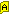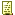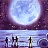Richard H.(KiX Supporter) 2004-11-03 04:58 PMUsing ADO to convert to / from BYTE arrays.

As you will know, KiXtart does not handle bytes which mean that manipulating binary objects (and/or files) is a bit of a problem.

Specifically I needed to convert a binary SID to it's string value.

To this end, I've created a series of UDFs which will allow the manipulation of byte arrays.

These UDFs require ADO 2.5 - I think that this is ubiquitous for Windows installations now, but if this is not the case then please let me know.

This example displays all groups and user with their associated SID:
Code:
```Break ON
\$=SetOption("Explicit","ON")
\$=SetOption("WrapAtEOL","ON")

Dim \$sDomain,\$oDomain,\$oGroup,\$sGroupPath,\$avSID,\$aiBinarySID,\$i

\$sDomain="YOUR_DOMAIN_HERE"
\$oDomain=GetObject("WinNT://"+\$sDomain)
\$oDomain.Filter="group",""
"GROUP SIDS: " ?
For Each \$oGroup In \$oDomain
\$avSID=\$oGroup.GetEx("objectSID")
\$aiBinarySID=udfBytes2Array(\$avSID)
\$oGroup.Name+", SID="+udfSID2String(\$aiBinarySID) ?
Next

\$oDomain.Filter="user",""
"USER SIDS: " ?
For Each \$oGroup In \$oDomain
\$avSID=\$oGroup.GetEx("objectSID")
\$aiBinarySID=udfBytes2Array(\$avSID)
\$oGroup.Name+", SID="+udfSID2String(\$aiBinarySID) ?
Next

\$oDomain=0
\$oGroup=0
Exit 0

; Convert a SID in binary format to SDDL format
; Note, I cheat here with the 48 bit authority value.
; It works though ;)
Function udfSID2String(\$aiSID)
Dim \$i,\$dAuth

\$udfSID2String="S-"+\$aiSID+"-"+\$aiSID+"-"+\$aiSID
For \$i = 12 To UBound(\$aiSID) Step 4
\$dAuth=CDbl(\$aiSid[\$i+3])
\$dAuth=\$dAuth*256+CDbl(\$aiSid[\$i+2])
\$dAuth=\$dAuth*256+CDbl(\$aiSid[\$i+1])
\$dAuth=\$dAuth*256+CDbl(\$aiSid[\$i+0])
\$udfSID2String=\$udfSID2String+"-"+CStr(\$dAuth)
Next
EndFunction
```

These are the binary / int conversion routines:
Code:
```; Convert an array of bytes to an array of integers
Function udfBytes2Array(\$abBytes)
Dim \$oStream,\$adTypeBinary,\$adTypeText,\$i

\$adTypeBinary=1

\$oStream=CreateObject("ADODB.Stream")
If @ERROR Exit @ERROR EndIf

\$oStream.Type=\$adTypeBinary
\$oStream.Open

\$oStream.Write(\$abBytes)
\$oStream.Position=0

Redim Preserve \$udfBytes2Array[\$oStream.Size-1]

For \$i = 0 To UBound(\$udfBytes2Array)
\$udfBytes2Array[\$i]=Asc(\$oStream.Read(1))
Next

\$oStream=0

Exit 0
EndFunction

; Convert an array of integers to an array of bytes
Function udfArray2Bytes(\$aiBytes,Optional \$iBytesToConvert)
Dim \$oStream,\$adTypeBinary,\$adTypeText,\$i

\$adTypeBinary=1
\$adTypeText=2

If Not \$iBytesToConvert \$iBytesToConvert=UBound(\$aiBytes)+1 EndIf

\$oStream=CreateObject("ADODB.Stream")
If @ERROR Exit @ERROR EndIf

\$oStream.Type=\$adTypeBinary
\$oStream.Open

For \$i = 1 To \$iBytesToConvert
\$oStream.Write(udfIntToByte(\$aiBytes[\$i-1]))
Next

\$oStream.Position=0
\$oStream.Type=\$adTypeBinary
\$udfArray2Bytes=\$oStream.Read(\$iBytesToConvert)

Exit 0
EndFunction

; Convert a single integer to a byte array
Function udfIntToByte(\$i)
Dim \$oStream,\$adTypeBinary,\$adTypeText

\$adTypeBinary=1
\$adTypeText=2

\$oStream=CreateObject("ADODB.Stream")
If @ERROR Exit @ERROR EndIf

\$i=CInt(\$i) & 255

\$oStream.Type=\$adTypeText
\$oStream.CharSet="unicode"
\$oStream.Open
\$oStream.WriteText(" "+Chr(\$i))
\$oStream.Position=0
\$oStream.Type=\$adTypeBinary

If \$i
\$oStream.Position=\$oStream.Size-2
Else
\$oStream.Position=\$oStream.Size-1
EndIf
\$udfIntToByte=\$oStream.Read(1)
Exit 0

EndFunction```

 Les (KiX Master) 2004-11-03 05:46 PMRe: Using ADO to convert to / from BYTE arrays.

Have you looked at my GetSID() UDF? It converts a binary SID to it's string value.

 Richard H.(KiX Supporter) 2004-11-04 09:43 AMRe: Using ADO to convert to / from BYTE arrays.

Yes I did and the Google trail that it started led to information that I used to create these UDFs , however your UDF requires a DLL from one of the SDKs and I was keen to avoid that.

The ADO requirement is more easy to fulfil.

Additionally, these routines allow the manipulation of any binary data in KiXtart - binary files, network IO and so-on. Anything that can be presented as a byte array.

 Richard H.(KiX Supporter) 2009-07-27 10:03 AMRe: Using ADO to convert to / from BYTE arrays.

Don't use these routines at present, one of them has a bug.

I just created a test suite which converts all 0-255 bytes to a byte array and then back again to a standard KiXtart integer arrary to make sure that the functions still work OK.

There is a problem with integers 128 - 159.

I'm not sure yet which of the routines is failing, though I've got a pretty good idead which one and why. I'll work on them as soon as I get some free time.

 Lonkero(KiX Master Guru) 2012-03-16 07:53 PMRe: Using ADO to convert to / from BYTE arrays.

int to byte?

 It_took_my_meds (Hey THIS is FUN) 2012-03-17 12:40 PMRe: Using ADO to convert to / from BYTE arrays.

Please check out Extract icon from resource file. There is a bytes.bin file that I created attached that you can use to convert int to byte using Adodb.Stream.

 PapaZod (Fresh Scripter) 2014-10-23 04:19 PMRe: Using ADO to convert to / from BYTE arrays.

This is a great thread and thanks for the ideas. One thing I noted is that often the token list is used for comparison to a group objectSID. This being the case, and that arrays are difficult to compare, I rewrote the udfs to produce strings and not arrays. This way you can simply do a single conversion, and compare the HEX strings instead of having to convert all the way to the standard SID format before comparison.

I've included the full script which uses the new "string" routines. This *should* run "As Is":

Code:
```\$ADS_NAME_TYPE_NT4 = 3
\$ADS_NAME_TYPE_1779 = 1

\$rootDSE = GetObject("LDAP://RootDSE")
\$DomainContainer = \$rootDSE.Get("defaultNamingContext")
\$DomainShortName = Split(Split(\$DomainContainer,","),"=")
\$CurrentUser = @USERID
\$NameTrans = CreateObject("nametranslate")
\$NameTrans.set(\$ADS_NAME_TYPE_NT4, \$DomainShortName + "\" + \$CurrentUser)
\$DN = \$NameTrans.get(\$ADS_NAME_TYPE_1779)
\$oUser = GetObject("LDAP://" + \$DN)
\$oUser.GetInfo
\$arrTG = "tokengroups",""
\$oUser.GetInfoEx(\$arrTG, 0)
\$GroupList = \$oUser.GetEx("tokengroups")

? "" + (ubound(\$GroupList)+1) + " entries returned"
?"Listing groups"?
For \$i = 0 to ubound(\$GroupList)
\$hexSID = OctetToHexStr(\$GroupList[\$i])
\$sSID = HexSID2DecString(\$hexSID)
\$bindSID = "LDAP://<SID=" + \$sSID + ">"
\$oObject = GetObject(\$bindSID)
if not @ERROR
\$Pad = ""
if len(\$sSID) < 47
for \$j = 1 to (47 - len(\$sSID))
\$Pad = \$Pad + " "
Next
endif
\$CN = \$oObject.Get("cn")
? \$sSID + " " + \$Pad + \$CN
endif
Next
?
Exit 0

Function HexSID2DecString(\$sSID)
; Convert a Hex string SID to the Decimal String representation
; S-1-5-21-....
Dim \$i, \$j, \$arrbytSID[], \$lngTemp

ReDim \$arrbytSID[(Len(\$sSID)/2) - 1]
For \$j = 0 To UBound(\$arrbytSID)
\$arrbytSID[\$j] = Val("&" + Substr(\$sSID, (2 * \$j) + 1, 2))
Next

\$HexSID2DecString = "S-" + CStr(\$arrbytSID) + "-" + CStr(\$arrbytSID) + "-" + CStr(\$arrbytSID)
For \$i = 12 To UBound(\$arrbytSID) Step 4
\$lngTemp = CDbl(\$arrbytSID[\$i + 3])
\$lngTemp = \$lngTemp * 256 + CDbl(\$arrbytSID[\$i + 2])
\$lngTemp = \$lngTemp * 256 + CDbl(\$arrbytSID[\$i + 1])
\$lngTemp = \$lngTemp * 256 + CDbl(\$arrbytSID[\$i])
\$HexSID2DecString = \$HexSID2DecString + "-" + CStr(\$lngTemp)
Next

EndFunction

Function OctetToHexStr(\$abBytes)
; Convert the SID byte array to a HEX
; string representation of the SID
;
; Uses ADO.Stream for data type conversion
; because kiXtart can't handle typed arrays

Dim \$oStream,\$adTypeBinary,\$i

\$adTypeBinary = 1
\$oStream = CreateObject("ADODB.Stream")

If @ERROR
? "error creating stream"
Exit @ERROR
EndIf
\$oStream.Type = \$adTypeBinary
\$oStream.Open

\$oStream.Write(\$abBytes)
\$oStream.Position=0

\$OctetToHexStr = ""
For \$i = 0 To \$oStream.size - 1
\$OctetToHexStr = \$OctetToHexStr + Right("0" + CStr(DecToHex(CDbl(Asc(\$oStream.Read(1))))),2)
Next

\$oStream=0
EndFunction
```